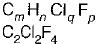Courses

# Refrigerants - 2

## 10 Questions MCQ Test Mock Test Series - Mechanical Engineering (ME) for GATE 2020 | Refrigerants - 2

Description
This mock test of Refrigerants - 2 for Mechanical Engineering helps you for every Mechanical Engineering entrance exam. This contains 10 Multiple Choice Questions for Mechanical Engineering Refrigerants - 2 (mcq) to study with solutions a complete question bank. The solved questions answers in this Refrigerants - 2 quiz give you a good mix of easy questions and tough questions. Mechanical Engineering students definitely take this Refrigerants - 2 exercise for a better result in the exam. You can find other Refrigerants - 2 extra questions, long questions & short questions for Mechanical Engineering on EduRev as well by searching above.
QUESTION: 1

Solution:
QUESTION: 2

Solution:
QUESTION: 3

### Which of the following has least starting torque requirement for appropriate sized compressors to produce the same refrigerating effect for different refrigerants?

Solution:

R1529, R134a, R600a demand higher starting torque motors than R12. If available R12 compressors of required larger displacements volumes are used with these substitutes, the starting torques of the motors could be inadequate, and starting problem might result. The motor mav trio constantly.

QUESTION: 4

In refrigeration system refrigerant gains heat at

Solution:

In refrigeration system, heat is absorbed at evaporator and rejected at condenser.

QUESTION: 5

Absorption system normally uses the following refrigerant

Solution:

In the absorption system of Aqua-Ammonia type Ammonia is used as a refrigerant and water is used as a absorbent. The Aqua-Ammonia absorption system is most popular type absorption system.

QUESTION: 6

The refrigerant used for absorption refrigerators working from solar collectors is a mixture of water and

Solution:

The another type of absorption system (lithium bromide absorption system), in which lithium bromide is used as absorbent and water is used as refrigerant.

QUESTION: 7

Which of the following is not a desirable characteristic of ideal absorbent in vapour absorption system?

Solution:
QUESTION: 8

Absorbent in a vapour absorption refrigerator system separates from the refrigerant only when it

Solution:

When the mixture of absorbent and refrigerant is heated sufficiently in the generator they get separate and refrigerant vapour is send to condenser and absorbent is sent back to the absorber.

QUESTION: 9

The number of hydrogen atoms in the refrigerant R-12 is

Solution:

R - 012
By comparing R-(m - 1)(n + 1) P
m - 1 = 0 ⇒ m = 1
n + 1 = 1 ⇒ n = 0
P = 2
where, n = number of hydrogen atom
m = number of carbon atom

QUESTION: 10

The correct nomenclature for ethylene is

Solution:

R-114
By comparing R - (m - 1) (n + 1)p
We get,
m - 1 = 1; ⇒ m = 2
n + 1 = 1
⇒ n = 0; p = 4
2m + 2 = n + p + q
6 = 0 + 4 + q ; q = 2
Hence its chemical formula isAlso, CF2Cl-CF2Cl